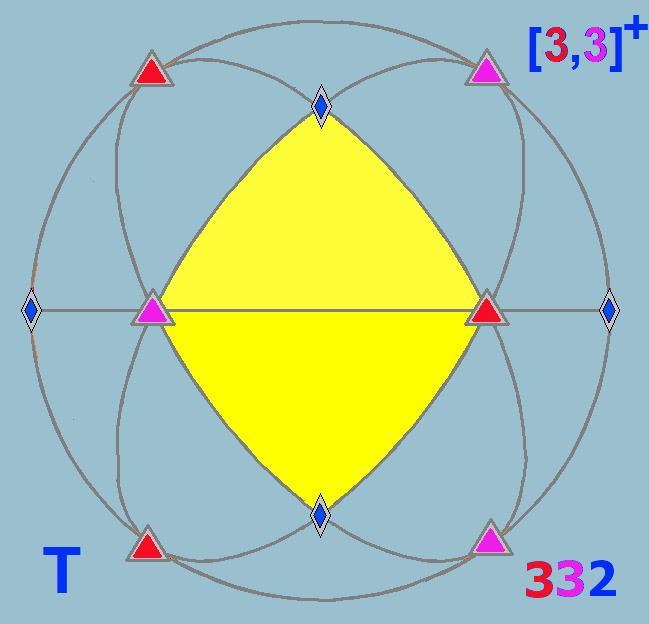# Polyhedral group

Updated on
Covid-19In geometry, the polyhedral group is any of the symmetry groups of the Platonic solids.

## Groups

There are three polyhedral groups:

• The tetrahedral group of order 12, rotational symmetry group of the regular tetrahedron.
• The conjugacy classes of T are:
• identity
• 4 × rotation by 120°, order 3, cw
• 4 × rotation by 120°, order 3, ccw
• 3 × rotation by 180°, order 2
• The octahedral group of order 24, rotational symmetry group of the cube and the regular octahedron.
• The conjugacy classes of O are:
• identity
• 6 × rotation by 90°, order 4
• 8 × rotation by 120°, order 3
• 3 × rotation by 180°, order 4
• 6 × rotation by 180°, order 2
• The icosahedral group of order 60, rotational symmetry group of the regular dodecahedron and the regular icosahedron.
• The conjugacy classes of I are:
• identity
• 12 × rotation by 72°, order 5
• 12 × rotation by 144°, order 5
• 20 × rotation by 120°, order 3
• 15 × rotation by 180°, order 2
• These symmetries double to 24, 48, 120 respectively for the full reflectional groups. The reflection symmetries have 6, 9, and 15 mirrors respectively. The octahedral symmetry, [4,3] can be seen as the union of 6 tetrahedral symmetry [3,3] mirrors, and 3 mirrors of dihedral symmetry Dih2, [2,2]. Pyritohedral symmetry is another doubling of tetrahedral symmetry.

The conjugacy classes of full tetrahedral symmetry, Td, are:

• identity
• 8 × rotation by 120°
• 3 × rotation by 180°
• 6 × reflection in a plane through two rotation axes
• 6 × rotoreflection by 90°
• The conjugacy classes of pyritohedral symmetry, Th, include those of T, with the two classes of 4 combined, and each with inversion:

• identity
• 8 × rotation by 120°
• 3 × rotation by 180°
• inversion
• 8 × rotoreflection by 60°
• 3 × reflection in a plane
• The conjugacy classes of the full octahedral group, Oh, are:

• inversion
• 6 × rotoreflection by 90°
• 8 × rotoreflection by 60°
• 3 × reflection in a plane perpendicular to a 4-fold axis
• 6 × reflection in a plane perpendicular to a 2-fold axis
• The conjugacy classes of full icosahedral symmetry Ih include also each with inversion:

• inversion
• 12 × rotoreflection by 108°, order 10
• 12 × rotoreflection by 36°, order 10
• 20 × rotoreflection by 60°, order 6
• 15 × reflection, order 2

Topics